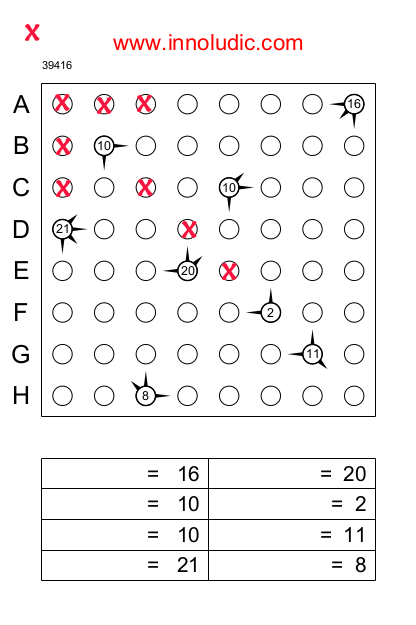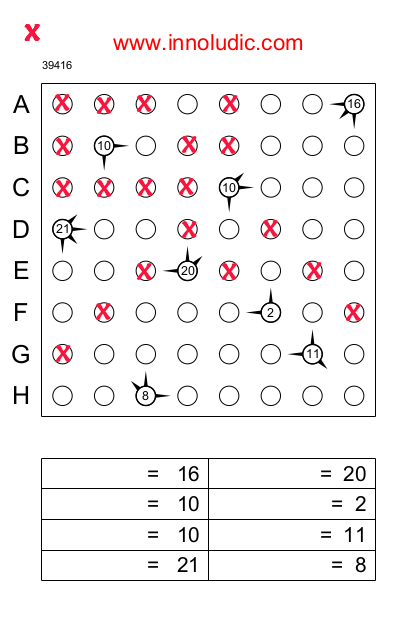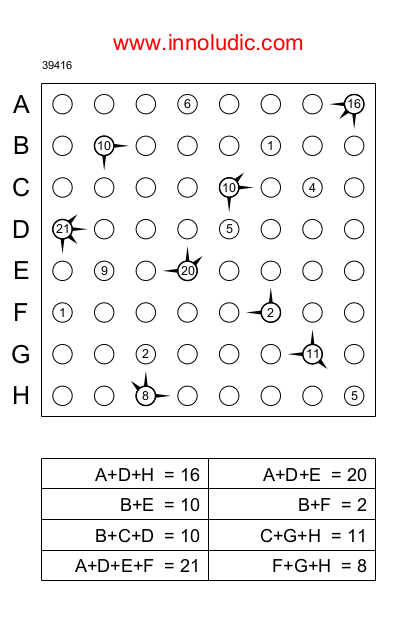Rules of the Space Ships

## Rules of the Space Ships

### The  Space Ships

Here is a game that is known in Spain as "nave". It's a game that appeals to your logical faculties as well as your mathematical abilities. For this reason, I find it attractive and interesting. I have adapted this game a little bit to my own way.### Here is the scenario:

The earth is invaded by alien ships.  To neutralize this attack, you have to find the location of these ships and their powers. In the grid there is one ship per row and column and each ship has a power from 1 to 9. To help you, there are radars (circles with numbers and arrows around them) which indicate the direction of the detected ships. The number in the radar indicates the total power of the detected ships.

When a direction is diagonal, the first ship on that diagonal is detected.  As each arrow on the radar points to a ship it also means that if a radar does not point in a direction it means that there is no ship between this radar and the end of the line (either vertical, horizontal or diagonal) or the next radar on this same line.

In short, you need to know where these ships are and how powerful they are.  I will solve the attached example to show you how to do this.### First Step; Find the Space Ships.

To find the places in the grid where the ships are, it is advisable to eliminate the places where the ships are not there.  For example, the radar at line B does not show any ships in the directions where there are no arrows. So we can mark these circles with X's.  Note the circles on the SOUTH-EAST diagonal, we stop eliminating the circles until the first radar we encounter. Moreover on this same diagonal the radar at line G indicates a vessel in this direction and there remains only one circle. So the radar should go in this circle. But for the purposes of the example, I do not identify it immediately. Here is the result of applying this technique for radar B.You should do the same thing for each radar, then it will become easier to find the ships in the remaining circles. Here are the results after the elimination of cells of the radar of the line C.We notice that the radar of the line E points towards the North and it remains only one free circle then bravo! we have just identified a ship which we put in blue.By continuing to cogitate we can identify the location of each space ship.### Second Step` Find the Powers.

Here is the first step accomplished it is now necessary to find the power of each ship. You can try by yourself to find the powers. But to make it easier, we will need some algebra (of a very limited level).  At the bottom of the grid, there is a table with the totals for each radar. Simply enter the line of the ship that is pointed by the radar. For example the first radar has a value of 16 and it points to the ships of the lines A, D and H. So we write A+D+H . We do the same thing for each radar and we should obtain the following table.Now you just have to look and compare carefully the equations in order to find a value. And then using the result found we continue to find another powers and so on we gradually manage to find all the powers.

For example we have an equation which gives:

A+D+E=20

And another one which gives:

A+D+E+F=21

We can conclude that F=1 and we can find B

Because B+F=2 and we know that F=1 so B=1

Finally after applying this several times, we find the solution.My adaptations were to add the equation table, to name each line (A, B, C, etc..) and especially to make sure that the system of equations is solvable.The game presented is of size 8x8 that is to say 8 ships and 8 equations, it is the easiest format. I have additional sizes, like  10x10 (medium level) and also more complicated level like 12x12, 14x14, 16x16 and 18x18.

Have fun  !.